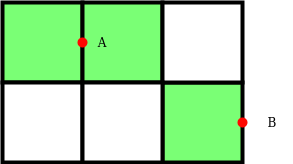# Minimum number of points required to cover all blocks of a 2-D grid

Given two integers N and M. The task is to find the minimum number of points required to cover an N * M grid.

A point can cover two blocks in a 2-D grid when placed in any common line or sideline.

Examples:

Input: N = 5, M = 7
Output: 18

Input: N = 3, M = 8
Output: 12

## Recommended: Please try your approach on {IDE} first, before moving on to the solution.

Approach: This problem can be solved using Greedy Approach. The main idea is to observe that a single point placed on the common line or sideline covers two blocks. So the total number of points needed to cover all the blocks(say B blocks) is B/2 when B is even else B/2 + 1 when B is odd.

For a grid having N*M blocks, The total number of blocks will be (N*M)/2 when either one of them is even. Otherwise, it will require ((N*M)/2) + 1 points to cover all the blocks and one extra for last untouched block.

Below is the image to show how points can be used to cover block in a 2D-grid:Point ‘A’ covers two blocks and ‘B’ covers one block.

Below is the implementation of the above approach:

## C++

 `// C++ program for the above approach ` `#include ` `using` `namespace` `std; ` ` `  `// Function to find the minimum number ` `// of Points required to cover a grid ` `int` `minPoints(``int` `n, ``int` `m) ` `{ ` `    ``int` `ans = 0; ` ` `  `    ``// If number of block is even ` `    ``if` `((n % 2 != 0) ` `        ``&& (m % 2 != 0)) { ` `        ``ans = ((n * m) / 2) + 1; ` `    ``} ` `    ``else` `{ ` `        ``ans = (n * m) / 2; ` `    ``} ` ` `  `    ``// Return the minimum points ` `    ``return` `ans; ` `} ` ` `  `// Driver Code ` `int` `main() ` `{ ` `    ``// Given size of grid ` `    ``int` `N = 5, M = 7; ` ` `  `    ``// Function Call ` `    ``cout << minPoints(N, M); ` `    ``return` `0; ` `} `

## Java

 `// Java program for the above approach ` `class` `GFG{ ` `     `  `// Function to find the minimum number ` `// of Points required to cover a grid ` `static` `int` `minPoints(``int` `n, ``int` `m) ` `{ ` `    ``int` `ans = ``0``; ` ` `  `    ``// If number of block is even ` `    ``if` `((n % ``2` `!= ``0``) && (m % ``2` `!= ``0``))  ` `    ``{ ` `        ``ans = ((n * m) / ``2``) + ``1``; ` `    ``} ` `    ``else`  `    ``{ ` `        ``ans = (n * m) / ``2``; ` `    ``} ` ` `  `    ``// Return the minimum points ` `    ``return` `ans; ` `} ` ` `  `// Driver Code ` `public` `static` `void` `main (String[] args)  ` `{ ` `    ``// Given size of grid ` `    ``int` `N = ``5``, M = ``7``; ` ` `  `    ``// Function Call ` `    ``System.out.print(minPoints(N, M)); ` `} ` `} ` ` `  `// This code is contributed by Ritik Bansal `

## Python3

 `# Python3 program for the above approach ` ` `  `# Function to find the minimum number  ` `# of Points required to cover a grid  ` `def` `minPoints(n, m): ` ` `  `    ``ans ``=` `0` ` `  `    ``# If number of block is even ` `    ``if` `((n ``%` `2` `!``=` `0``) ``and` `(m ``%` `2` `!``=` `0``)): ` `        ``ans ``=` `((n ``*` `m) ``/``/` `2``) ``+` `1` ` `  `    ``else``: ` `        ``ans ``=` `(n ``*` `m) ``/``/` `2` ` `  `    ``# Return the minimum points ` `    ``return` `ans ` ` `  `# Driver code ` `if` `__name__ ``=``=` `'__main__'``: ` ` `  `    ``# Given size of grid ` `    ``N ``=` `5` `    ``M ``=` `7` ` `  `    ``# Function call ` `    ``print``(minPoints(N, M)) ` ` `  `# This code is contributed by himanshu77 `

Output:

```18
```

Time Complexity: O(1)
Auxiliary Space: O(1)

Attention reader! Don’t stop learning now. Get hold of all the important DSA concepts with the DSA Self Paced Course at a student-friendly price and become industry ready.

My Personal Notes arrow_drop_upCheck out this Author's contributed articles.

If you like GeeksforGeeks and would like to contribute, you can also write an article using contribute.geeksforgeeks.org or mail your article to contribute@geeksforgeeks.org. See your article appearing on the GeeksforGeeks main page and help other Geeks.

Please Improve this article if you find anything incorrect by clicking on the "Improve Article" button below.

Improved By : btc_148, himanshu77